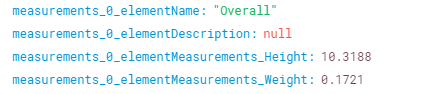# Generic function to flatten nested JSON as input to Retool component

Hi there. This really isn't a Retool thing but finally managed to make a function to flatten nested JSON as an input to a Retool component. Very often I'm hand-crafting deep object references, and then using map to parse an array in an object etc, when really I just want to extract a handful of items from a few different levels in the hierarchy.

Example use
For this demo I'm pulling a random entry from the met museum api (doesn't require any authentication):

The api returns some nested json, which can change very significantly depending upon which object is returned (like many APIs):

Which is then 'flattened' to this:

This is the JS query which does the conversion:

``````const x_data = any_api.data

function parse_object(x_input_object, x_input_path) {

let x_flat_object = {}
let x_curr_path = ''

if (x_input_path.length > 0 ) {
x_curr_path = x_input_path + '_'
}
else {
x_curr_path = '' }

let x_this_obj_entries = Object.entries(x_input_object)

x_this_obj_entries.forEach((x_entry) => {
if (typeof x_entry !== 'object' || x_entry === null) {
x_flat_object[x_curr_path + x_entry] = x_entry
}
else {
let x_recursive_object = parse_object(x_entry, x_curr_path + x_entry)
Object.assign(x_flat_object, x_recursive_object)
}
})
return x_flat_object
}

var x_output = parse_object(x_data, '')

return x_output
``````

## Behaviour

General
At each level in hierarchy it concatenates the path using an underscore
Arrays
When it meets an array, it concatenates the index of the array.
From:to:Objects
Appends the nested object name to the parent object name, basically dot notation but replacing the dot with an underscore.

In this example there is a top level property (measurements) which contains an array of objects. Those objects then have nested objects as properties (elementMeasurements), so you go from this:to this:If desired you can then throw this straight into a table:Now it is in one list, you can do things like filtering the list, in this case for anything with image in the name:

and then show those items:

Not the most concrete use case but the flattened list can be a lot easier to work with.

Hope that's of use!

2 Likes

Thanks so much for sharing this detailed example!I like this, but in case you just want to access some nested JSON data in a table, you can do it without any transformer. The table component has a mapping field that gives you access to sub-fields in the query.

1 Like